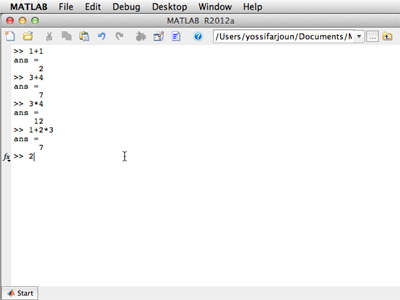18.S997 | Fall 2011 | Undergraduate

# Introduction To MATLAB Programming

## The BasicsMATLAB’s command prompt can be used for quick and easy calculations.

In this unit, you will learn how to use the MATLAB® command prompt for performing calculations and creating variables. Exercises include basic operations, and are designed to help you get familiar with the basics of the MATLAB interface. One of MATLAB’s conveniences is its ability to work with lists of numbers. You will have the opportunity to practice constructing and manipulating lists, vectors, and matrices. Since the unit also serves as an introduction to programming, you will receive guidance on defining variables, storing values in variables, and changing the values of variables.

The videos below demonstrate, step-by-step, how to work with MATLAB in relevance to the topics covered in this unit.